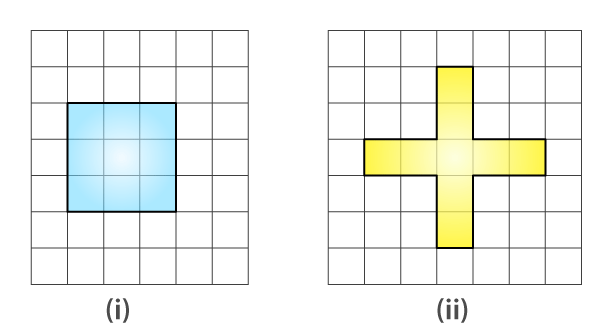# Avneet buys 9 square paving slabs, each with a side of 1 / 2 m. He lays them in the form of a square. (a) What is the perimeter of his arrangement [fig 10.7(i)]? (b) Shari does not like his arrangement. She gets him to lay them out like a cross. What is the perimeter of her arrangement [(Fig 10.7 (ii)]? (c) Which has greater perimeter? (d) Avneet wonders if there is a way of getting an even greater perimeter. Can you find a way of doing this? (The paving slabs must meet along complete edges i.e they cannot be broken.)Solutions:

(a)Side of square = 3 × side

= 3 × 1 / 2

= 3 / 2 m

Perimeter of Square = 4 × 3 / 2

= 2 × 3

= 6 m

(b) Perimeter = 0.5 + 1 + 1 + 0.5 + 1 + 1 + 0.5 + 1 + 1 + 0.5 + 1 + 1

= 10 m

(c) The arrangement given in the form of cross has greater perimeter

(d) Perimeters greater than 10 m cannot be determined.(1)(0)